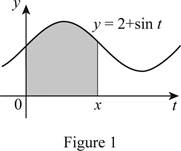# The g ′ ( x ) , using part 1 of fundamental theorem of calculus and integral evaluation.### Single Variable Calculus: Concepts...

4th Edition
James Stewart
Publisher: Cengage Learning
ISBN: 9781337687805### Single Variable Calculus: Concepts...

4th Edition
James Stewart
Publisher: Cengage Learning
ISBN: 9781337687805

#### Solutions

Chapter 5.4, Problem 6E

a)

To determine

## To calculate: The g′(x), using part 1 of fundamental theorem of calculus and integral evaluation.

Expert Solution

The g(x) value is 2+sinx_ by using part 1 of fundamental theorem of calculus method.

### Explanation of Solution

Given information:

g(x)=0x(2+sint)dt

Calculation:

Show the integral function as shown below:

g(x)=0x(2+sint)dt (1)

Here, g(x) is function of x andt is a derivative.

Draw the area represented g(x) as below;Show the part 1 of fundamental theorem of calculus as shown below:

g(x)=axf(t)dt (2)

g(x)=ddxaxf(t)dt (3)

Comparing Equation (1), (2) and (3).

g(x)=0x(2+sint)dtg(x)=f(x)=2+sinx.

Therefore, get the value of g(x) as 2+sinx_ by using the part 1 of fundamental theorem of calculus.

(b)

To determine

### The value for g′(x) by using the integral evaluation method.

Expert Solution

The g(x) value is 2+sinx_ by using the integral evaluation method.

### Explanation of Solution

Given information:

g(x)=0x(2+sint)dt

Calculation:

Apply the integral evaluation method:

g(x)=0x(2+sint)dt=(2tcost)0x=[(2xcosx)(0cos0)]=2xcosx+1.

Differentiate with respect to x.

g(x)=2xcosx+1g(x)=2(1)(sinx)=2+sinx.

Therefore, get the value of g(x) as 2+sinx_ using the integral method.

### Have a homework question?

Subscribe to bartleby learn! Ask subject matter experts 30 homework questions each month. Plus, you’ll have access to millions of step-by-step textbook answers!